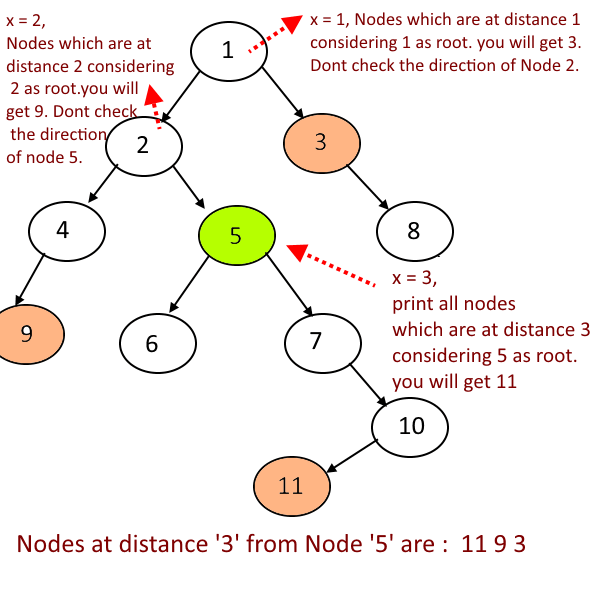Hard

# Print All The Nodes Which are X distance from the Given Node

Objective: - Given Binary Tree, Print All The Nodes Which are X distance from the Given Node.

Example :

Approach:

Quite Tricky solution, i will explain using the example given in the picture.
Find out all the nodes which are at distance '3' from the given node 5.

1. Find the height of the given node 5 is -3. say x = 3.
2. For all the nodes which are at distance 3 and exist below node 5, consider 5 as root node and problem will reduce to "Print all the nodes which are at distance x from the root". Agree?.
3. But what about the other nodes, go one level up ( at the path from root to node 5), which is node 2. since you have gone up by one level, x will be x = x-1 = 3-1 = 2.
4. So now consider node 2 as root and again problem will reduce to, Print all the nodes which are at distance 2 from the root. remember one thing when considering the node 2 as root, you dont check the direction of node 5 .(Why,?? because if you check nodes at distance 2 from node 2, you will get 9, 7. Node 9 is at distance 3 from Node 5 which is right but Node 7 is at distance 1 from Node 5 which is wrong.)
5. How would you stop your program to check in the direction of Node 5, when you go one level up, means at Node 2, after processing the Node 5, return Node 5 to Node 2 and at Node 2 check whether Node 5 is right child or left child of Node 2, based on that don't check that direction.
6. Now recursivley go one more level up, now x = x-1 = 2-1 =1. It will be Node 1, consider Node 1 as root and again problem will reduce to, Print all the nodes which are at distance 1 from the root and don't check the direction of Node 2, with the same logic explained earlier.Code:

Output:

`Nodes at distance '3' from Node '5' are : 11 9 3`